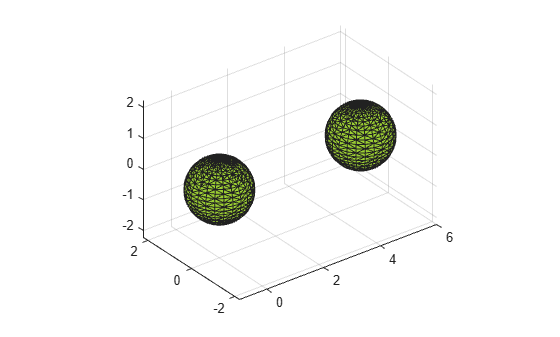# alphaTriangulation

Triangulation that fills alpha shape

## Syntax

``tri = alphaTriangulation(shp)``
``tri = alphaTriangulation(shp,RegionID)``
``````[tri,P] = alphaTriangulation(___)``````

## Description

example

````tri = alphaTriangulation(shp)` returns a triangulation that defines the domain of the alpha shape. Each row in `tri` specifies a triangle or tetrahedron defined by vertex IDs (the row numbers of the `shp.Points` matrix).```
````tri = alphaTriangulation(shp,RegionID)` returns a triangulation for a region of the alpha shape. `RegionID` is the ID for the region and `1` ≤ `RegionID` ≤ `numRegions(shp)`.```
``````[tri,P] = alphaTriangulation(___)``` also returns a matrix of vertex coordinates, `P`, using any of the previous syntaxes.```

## Examples

collapse all

Create a set of 3-D points.

```[x1, y1, z1] = sphere(24); x1 = x1(:); y1 = y1(:); z1 = z1(:); x2 = x1+5; P = [x1 y1 z1; x2 y1 z1]; P = unique(P,'rows');```

Create and plot an alpha shape for the point cloud using an alpha radius of 1.

```shp = alphaShape(P,1); plot(shp)```Use `alphaTriangulation` to recover the triangulation that defines the domain of the alpha shape.

`tri = alphaTriangulation(shp);`

Find the total number of tetrahedra that make up the alpha shape.

`numtetrahedra = size(tri,1)`
```numtetrahedra = 3755 ```

## Input Arguments

collapse all

Alpha shape, specified as an `alphaShape` object. For more information, see `alphaShape`.

Example: `shp = alphaShape(x,y)` creates a 2-D `alphaShape` object from the `(x,y)` point coordinates.

ID number for region in alpha shape, specified as a positive integer scalar between `1` and `numRegions(shp)`.

An alpha shape can contain several smaller regions, depending on the point set and parameters. Each of these smaller regions is assigned a unique `RegionID`, which numbers the regions from the largest area or volume to the smallest. For example, consider a 3-D alpha shape with two regions. The region with the largest volume has a `RegionID` of 1, and the smaller region has a `RegionID` of 2.

Example: `shp.RegionThreshold = area(shp,numRegions(shp)-2);` suppresses the two smallest regions in 2-D alpha shape `shp`.

Data Types: `double`

## Output Arguments

collapse all

Triangulation, returned as a matrix. `tri` is of size `mtri`-by-`nv`, where `mtri` is the number of triangles or tetrahedra in the alpha shape and `nv` is the number of vertices. The value of `nv` is `3` for 2-D alpha shapes and `4` for 3-D alpha shapes.

The number of outputs you specify with `alphaTriangulation` can change the vertex indexing used in `tri`.

Vertex coordinates, returned as a matrix. `P` is of size `N`-by-`dim`, where `N` is the number of points in the alpha shape and `dim` is either `2` or `3` (for either a 2-D or 3-D alpha shape).

## Version History

Introduced in R2014b